#### WATCH ALL SLIDES

Slide 1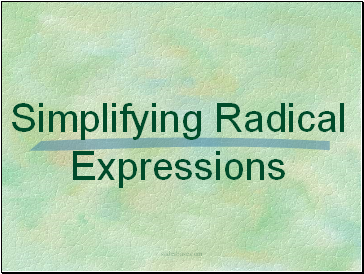Slide 2Slide 3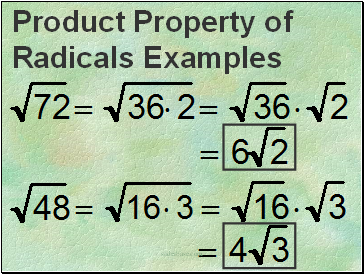Slide 4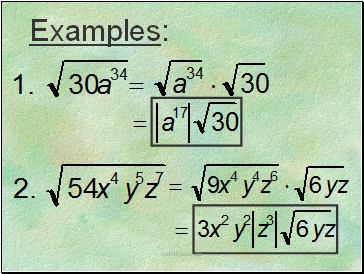Examples:

Slide 5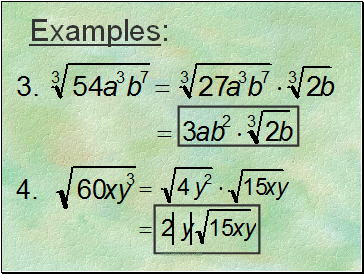Examples:

Slide 6Slide 7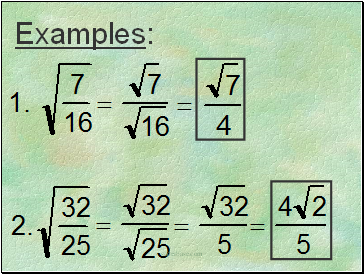Examples:

Slide 8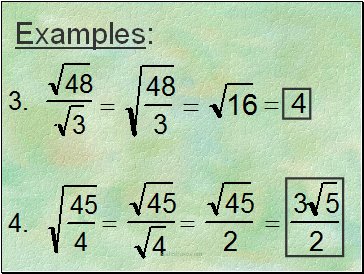Examples:

Slide 9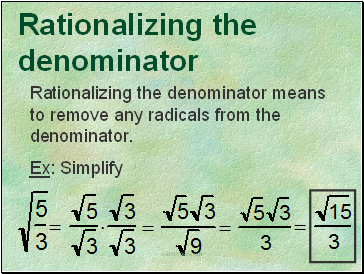## Rationalizing the denominator

Rationalizing the denominator means to remove any radicals from the denominator.

Ex: Simplify

Slide 10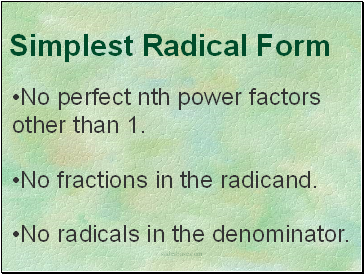No perfect nth power factors other than 1.

Slide 11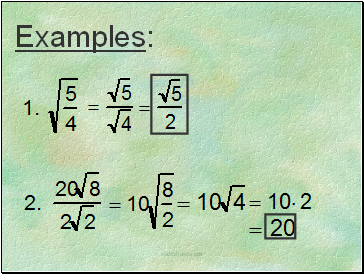Examples:

Slide 12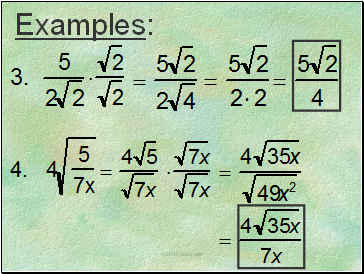Examples:

Slide 13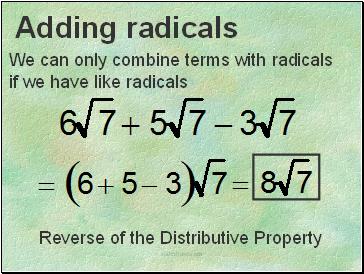We can only combine terms with radicals

Reverse of the Distributive Property

Slide 14Examples:

Slide 15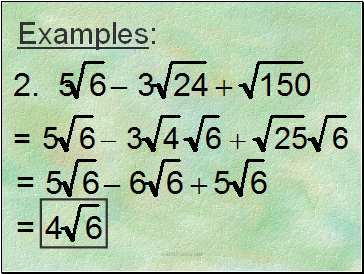Examples:

Slide 16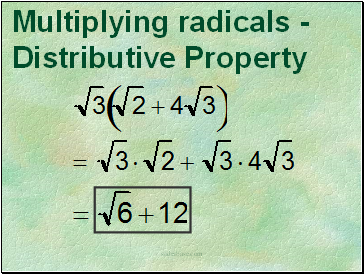Slide 17Slide 18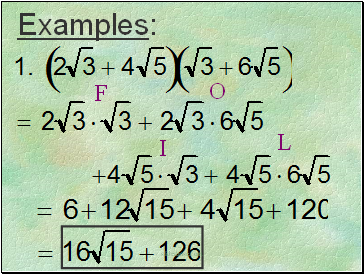Examples:

Slide 19Examples:

Slide 20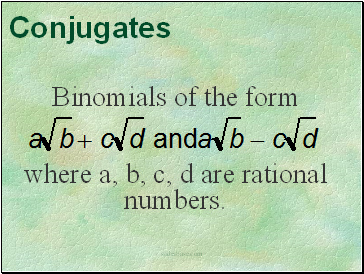Conjugates

Slide 21The product of conjugates is a rational number. Therefore, we can rationalize denominator of a fraction by multiplying by its conjugate.

Go to page:
1  2